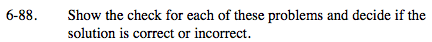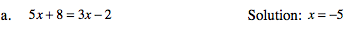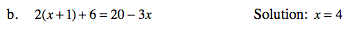### Home > MC2 > Chapter 6 > Lesson 6.2.2 > Problem6-88

6-88.Substitute the value in for x and solve to see if the two sides are equal to each other.

5 (−5) + 8 = 3 (−5) −2
−25 + 8 = −15 − 2

−17 = −17
Solution x = −5 is correct.See part (a).